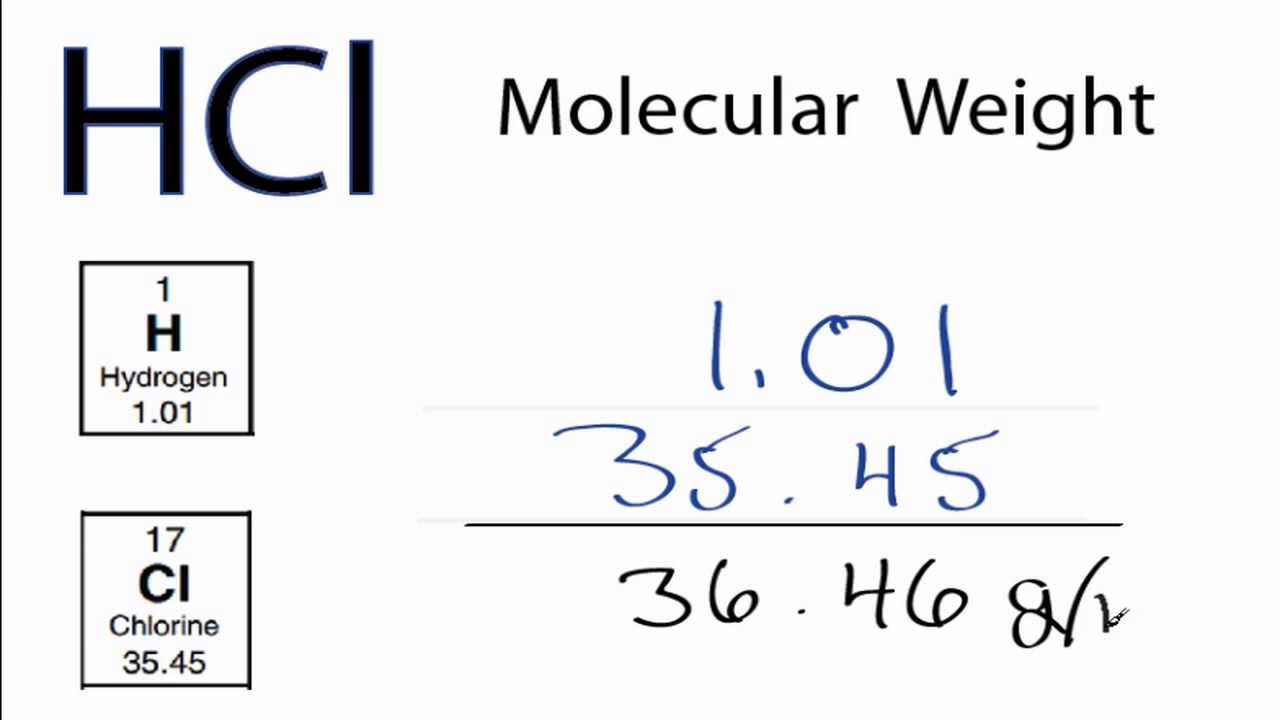# HCL Molar Mass: Unraveling the Secrets of Hydrochloric AcidHCL Molar Mass: Hydrochloric acid, commonly known as HCl, is an essential chemical compound with numerous industrial and biological applications. Understanding its properties, including its molar mass, is crucial for various scientific fields. This article delves into the world of HCl, exploring its composition, molar mass, and significance in different sectors. So, let’s embark on this informative journey and unravel the secrets of hydrochloric acid’s molar mass.

## I. Understanding Hydrochloric Acid:

Hydrochloric acid, denoted by its chemical formula HCl, is a strong, corrosive acid. It is a colorless liquid with a pungent smell and is highly soluble in water. HCl is widely used in various industries, including chemical manufacturing, metal processing, pharmaceuticals, and food production. Moreover, it plays a vital role in the human digestive system, aiding the breakdown of food in the stomach.

## II. Defining Molar Mass:

Molar mass refers to the mass of one mole of a substance and is expressed in grams per mole (g/mol). It is an essential concept in chemistry as it provides a quantitative measure of the amount of a substance present. Molar mass plays a crucial role in various chemical calculations, such as determining the number of moles or mass of a substance in a given sample.

## III. Calculating HCl Molar Mass:

To determine the molars mass of hydrochloric acid (HCl), we need to consider the atomic masses of hydrogen (H) and chlorine (Cl). Hydrogen has an atomic mass of approximately 1.01 g/mol, while chlorine has an atomic mass of around 35.45 g/mol. Since HCl consists of one hydrogen atom and one chlorine atom, we can add these atomic masses together to find the molars mass of HCl.

Thus, the molars mass of HCl = 1.01 g/mol (hydrogen) + 35.45 g/mol (chlorine) = 36.46 g/mol.

The molar mass of hydrochloric acid is approximately 36.46 grams per mole. This value helps in various chemical calculations, such as determining the amount of HCl required in a reaction or analyzing the concentration of HCl in a solution.

## IV. Significance of HCl Molar Mass:

The molar mass of hydrochloric acid holds great significance in different scientific and industrial applications. In the pharmaceutical industry, it is crucial to determine the precise dosage of HCl in medications. Additionally, knowing the molar mass aids in calculating the concentration of HCl in solutions used for chemical analysis.

Furthermore, understanding the molar mass of HCl is vital in controlling industrial processes. In metal processing, HCl is used for pickling and etching metals, where the molar mass helps determine the appropriate amount of acid required for efficient results. Similarly, in the food industry, molar mass calculations are essential for ensuring accurate measurements of HCl in food preservation and flavor enhancement processes.

## V. Conclusion:

Hydrochloric acid (HCl) is a versatile and vital compound used in various industries and biological processes. Understanding the molars mass of HCl is crucial for scientific calculations, dosage determination, and process control. With a molars mass of approximately 36.46 g/mol, HCl plays a significant role in chemical analysis, metal processing, pharmaceuticals, and food production. By unraveling the secrets of HCl molar mass, we gain valuable insights into the applications and importance of this remarkable compound.Previous post Broward CleverNext post Trixie Tounge Tricks